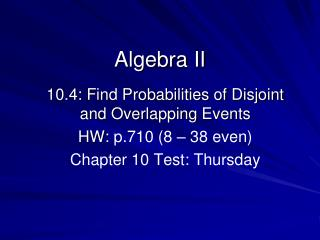DownloadDownload PresentationAlgebra II

# Algebra II

Download Presentation## Algebra II

- - - - - - - - - - - - - - - - - - - - - - - - - - - E N D - - - - - - - - - - - - - - - - - - - - - - - - - - -
##### Presentation Transcript

1. Algebra II 10.4: Find Probabilities of Disjoint and Overlapping Events HW: p.710 (8 – 38 even) Chapter 10 Test: Thursday

2. Overlapping EventsTwo events are overlapping if they have one or more outcomes in common.P(A or B) = P(A) + P(B) – P(A and B)

3. Disjoint EventsTwo events are disjoint, or mutually exclusive, if they have no outcomes in common. P(A or B) = P(A) + P(B) B A

4. Events A and B are disjoint. Find P(A or B). 1.) P(A) = 0.2, P(B) = 0.3 2.) P(A) = 34%, P(B) = 45%

5. Find the indicated probability. 1.) P(A) = 47%, P(B) = 21%, P(A or B) = 0.25, P(A and B) = ____________. 2.) P(A) = 0.25, P(B) = 0.32, P(A or B) = 0.45, P(A and B) = ____________.

6. A card is randomly drawn from a standard deck of 52 cards. Find the probability of drawing the indicated card. 1.) A heart or a diamond 2.) A spade or an even card

7. A card is randomly drawn from a standard deck of 52 cards. Find the probability of drawing the indicated card. 3.) A 6 or a 7 4.) An ace or a spade

8. A card is randomly drawn from a standard deck of 52 cards. Find the probability of drawing the indicated card. 5.) An ace and a spade 6.) A face card and a 4 7.) A spade and an even card

9. Algebra II 10.5: Find Probabilities of Independent and Dependent Events HW: worksheet

10. A jar contains 10 blue, 4 red,&6 white marbles. 1.) Find the probability of choosing a red, then a white marble with replacement. 2.) Find the probability of choosing a red, then a white marble without replacement.

11. A jar contains 10 blue, 4 red,&6 white marbles. 3.) Three marbles are chosen with replacement, find the probability that they are not white.

12. A jar contains 10 blue, 4 red,&6 white marbles. 4.) Three marbles are chosen without replacement, find the probability where at least one marble is white.

13. Find the probability of drawing the given cards from a standard deck of 52 cards (a) with replacement and (b) without replacement. 1.) a heart, then a spade

14. Find the probability of drawing the given cards from a standard deck of 52 cards (a) with replacement and (b) without replacement. 2.) a king, then a queen

15. Find the probability of drawing the given cards from a standard deck of 52 cards (a) with replacement and (b) without replacement. 3.) a 10, then a 10, then another 10

16. Find the probability of drawing the given cards from a standard deck of 52 cards (a) with replacement and (b) without replacement. 4.) a 4, then a 3, then a 2